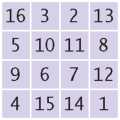Magic squares. An n × n matrix that is filled with the numbers 1, 2, 3, … , n2 is a magic square if the sum of the elements in each row, in each column, and in the two diagonals is the same value. For example,Write a program that reads in n2 values from the keyboard and tests whether they form a magic square when arranged as a square matrix. You need to test three features:
• Did the user enter n2 numbers for some n?

• Do each of the numbers 1, 2, … , n2 occur exactly once in the user input?

• When the numbers are put into a square, are the sums of the rows, columns, and diagonals equal to each other?

If the size of the input is a square, test whether all numbers between 1 and n2 are present. Then compute the row, column, and diagonal sums. Implement a class Square with methods

public boolean isMagic()

Complete the following file:

### Square.java

Use the following file:

MagicSquareChecker.java

```import java.util.ArrayList;
import java.util.Scanner;

/**
This class tests whether a sequence of inputs forms a magic square.
*/
public class MagicSquareChecker
{
public static void main(String[] args)
{
Scanner in = new Scanner(System.in);
Square sq = new Square();

System.out.println("Enter a sequence of integers, followed by Q: ");
while (in.hasNextInt())
{